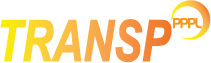The radiated power is used in the electron energy balance equation. Radiated power can be given to TRANSP as an input in the form of integrated power or as a profile. Alternatively, TRANSP can calculate the power due to bremsstrahlung, impurity line radiation and cyclotron radiation and use this in the energy balance.

The following namelist settings control how the radiation is included in the energy balance equations.

No radiation losses in the energy balance.
Use data from PREBOL/EXTBOL UFILE, rescale according to PRFAC if provided. No calculation of radiated power is undertaken in this case.
If the temperature profile is from experiments, then TRANSP uses data from PREBOL/EXTBOL.
If the temperature profile is predicted, then TRANSP uses the calculated radiated power.
This is the default setting in TRANSP.
Always use the calculated radiated power.

NOTE: TRANSP calculates by default (unless one of the settings above is selected) the total radiated power

The radiation for individual impurities can be calculated by setting the following flags: